+

# Case Study: Depreciation

Author: Sophia Tutorial
##### Description:

Calculate depreciation values using the straight line depreciation or double declining balance depreciation method.

(more)### Developing Effective Teams

*No strings attached. This college course is 100% free and is worth 1 semester credit.

37 Sophia partners guarantee credit transfer.

299 Institutions have accepted or given pre-approval for credit transfer.

* The American Council on Education's College Credit Recommendation Service (ACE Credit®) has evaluated and recommended college credit for 32 of Sophia’s online courses. Many different colleges and universities consider ACE CREDIT recommendations in determining the applicability to their course and degree programs.

Tutorial
what's covered
This lesson will cover performing a depreciation calculation using several different methods, in the context of a case study using a hypothetical company.

Our discussion breaks down as follows:

1. Case Study: Legacy Clothing
2. Case Study: Straight Line Depreciation
1. Buildings
2. Equipment
3. Case Study: Double Declining Balance Depreciation
1. Buildings
2. Equipment

## 1. Case Study: Legacy Clothing

The subject company for our case study is called Legacy Clothing. Legacy Clothing is a sole proprietorship, which is a type of company that is owned by a single individual, and where that individual and the business are legally treated as the same.

The purpose of Legacy Clothing as a business is to own and operate clothing/merchandise stores. It is similar to a department store chain, selling men's, women's, and children's clothing and other related items.

Legacy Clothing has locations throughout Washington, DC, and they have a staff of 50 people employed in their stores.

Legacy Clothing
Type of company Sole proprietorship
Business purpose Own and operate clothing/merchandise stores
Staff of 50 people

Legacy Clothing needs to calculate depreciation. As a company, they have long-term assets such as buildings, equipment, etc. Those assets are used over multiple periods, so those assets provide benefits to multiple periods.

Legacy Clothing needs to allocate the cost of those assets over those multiple usage periods. This allows them to achieve matching--the matching of cost and benefits. They are matching the cost of the asset through depreciation expense, and the benefit being received through revenue--in other words, matching expense with revenue.

## 2. Case Study: Straight Line Depreciation

Now let's look at performing some depreciation calculations for our subject company, Legacy Clothing, starting with a straight line depreciation calculation. The information we need to know in order to perform straight line depreciation is our total cost, residual value, as well as the useful life.

As you can see in the spreadsheet below, the formula for straight line depreciation is cost minus residual value divided by the number of years of the useful life.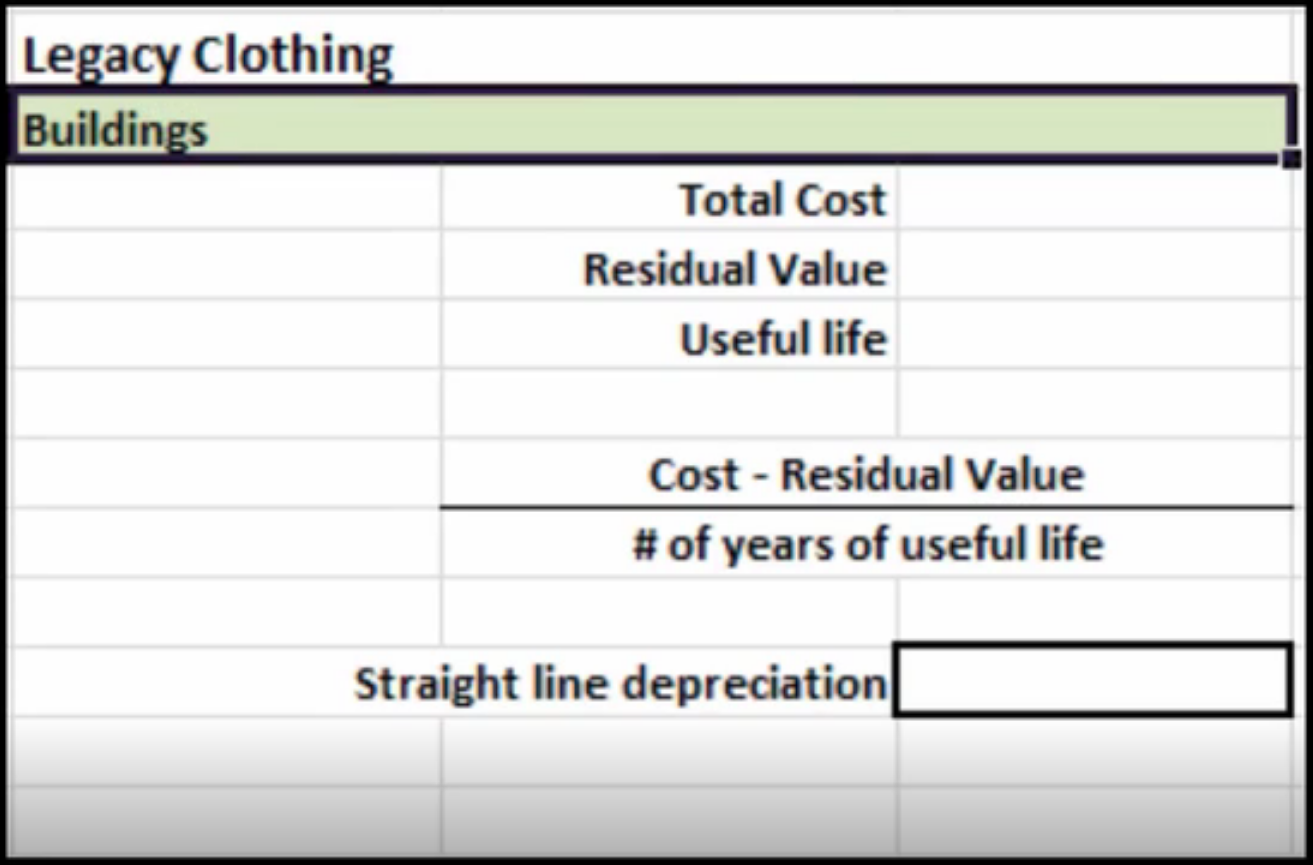2a. Buildings
The first calculation that we're going to perform is straight line depreciation on the asset buildings. So, let's go ahead and put the information in for our subject company.

We have buildings with a cost of \$500,000, and we assume there is no residual value for those buildings. We're also going to assume those buildings have a useful life of 30 years.

Plugging in this information--cost minus residual value divided by the number of years of useful life--we get our straight line depreciation of \$16,667. This is going to be our depreciation expense every year under straight line depreciation.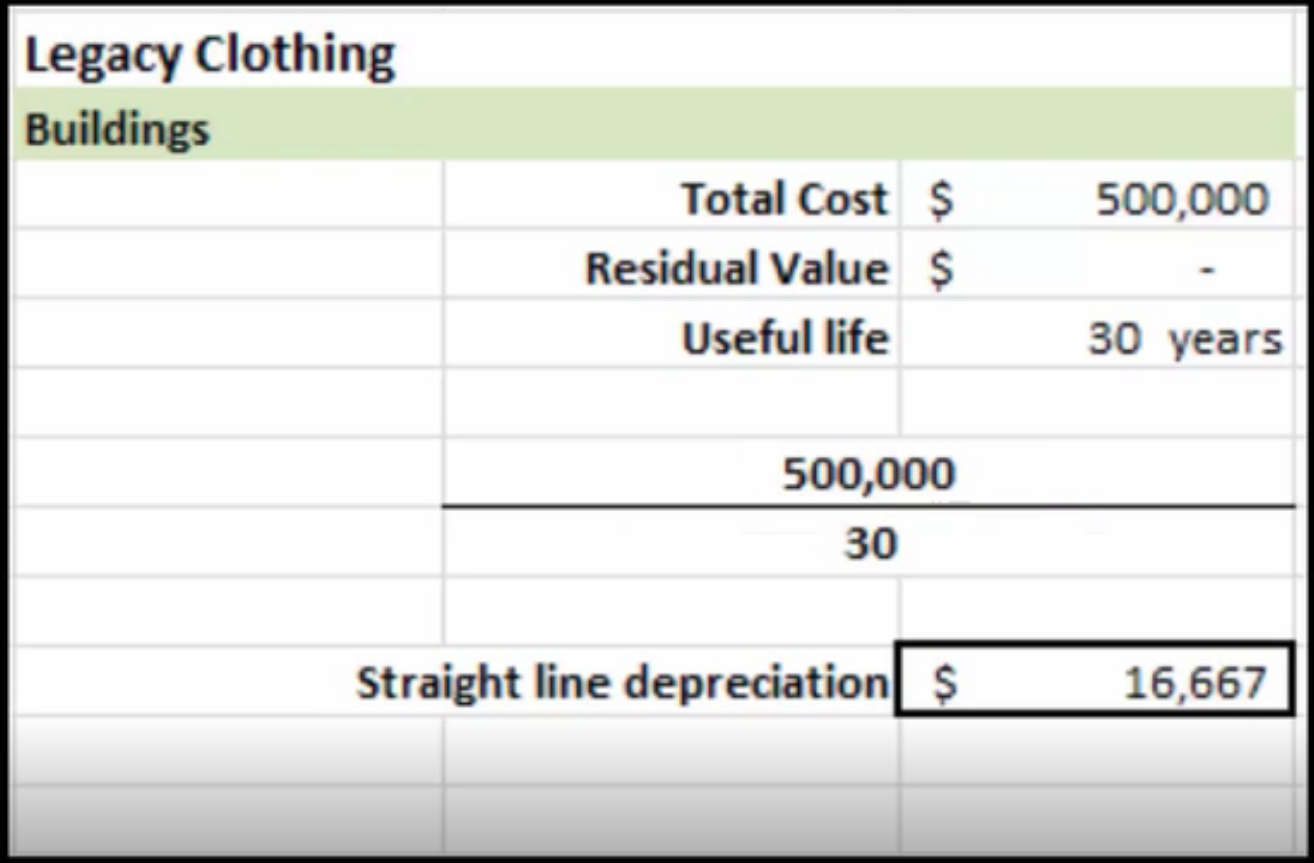Now, we can break our our annual depreciation expense into monthly depreciation by dividing by 12, the number of months in a year.

Also, if we wanted to know the total accumulated depreciation in any point throughout this asset's life, we can look at a specific number of months. For instance, in this case, after 60 months, or five years, our total accumulated depreciation is going to be \$83,333, which is our monthly depreciation multiplied by 60.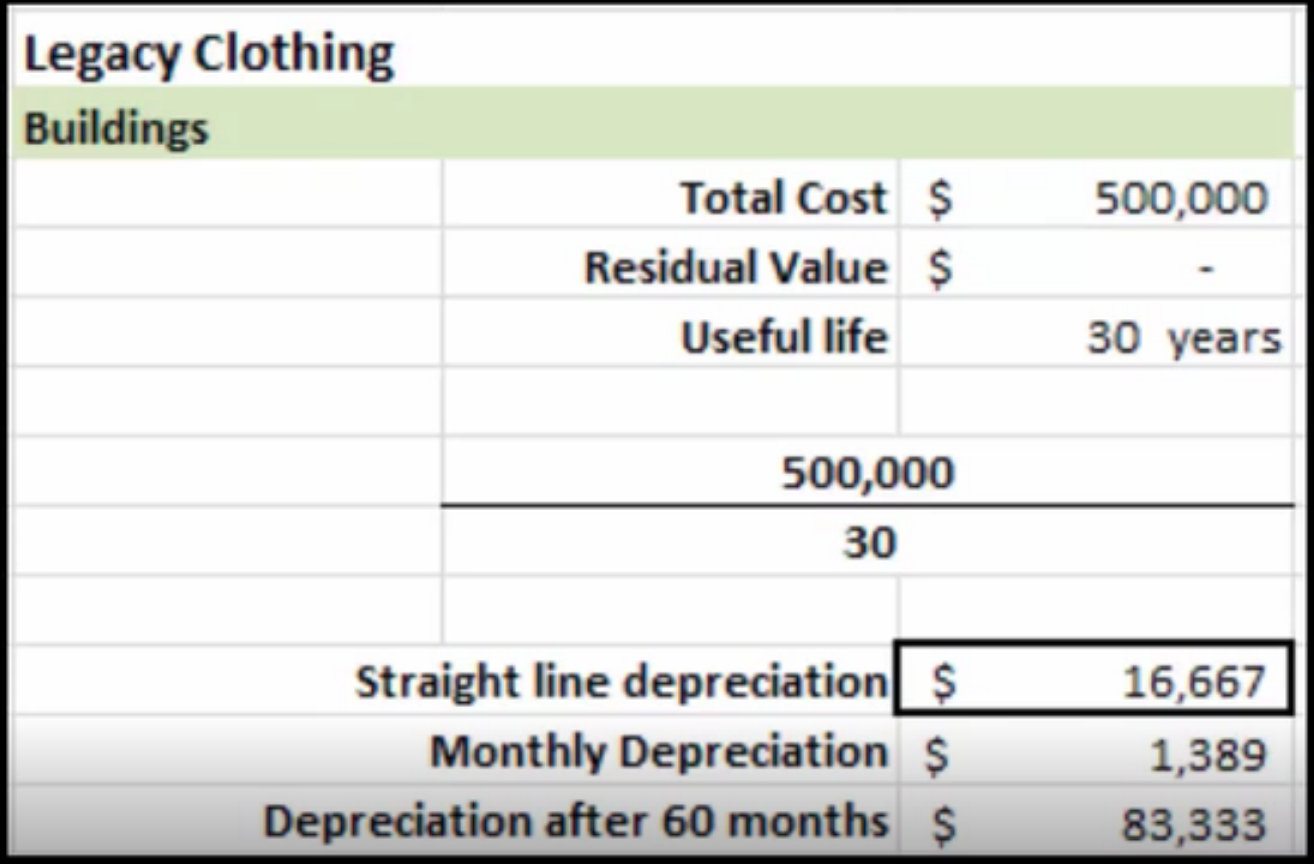2b. Equipment
Now we're going to perform the same straight line calculation, but in this case, for equipment, another fixed asset that we need to depreciate. Again, we need to know total cost, residual value, and the useful life.

We have \$200,000 worth of equipment and a residual value of \$10,000 for that equipment. That equipment has a useful life of 10 years.

So, if we look at our straight line depreciation formula, cost minus residual value divided by the number of years of useful life, we can plug in that information, \$190,000 divided by 10. This means that our straight line depreciation every year is going to be \$19,000.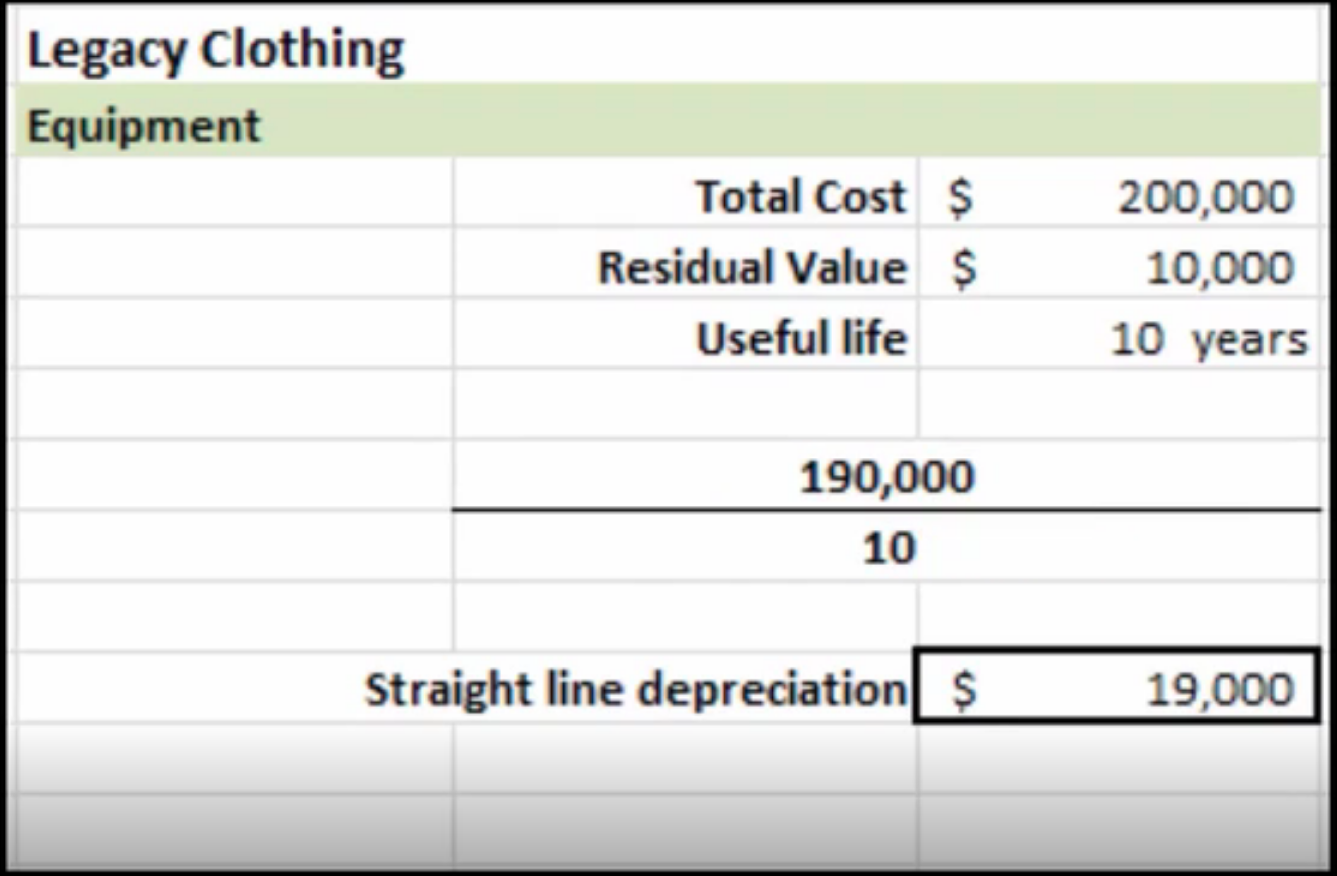Again, we can break that amount into monthly depreciation by dividing the straight line number by 12.

In addition, we can figure out the total accumulated depreciation at any point in time by plugging in the number of months that we're looking for. So, after 48 months, if we take our monthly depreciation and multiply it by 48, we would get \$76,000.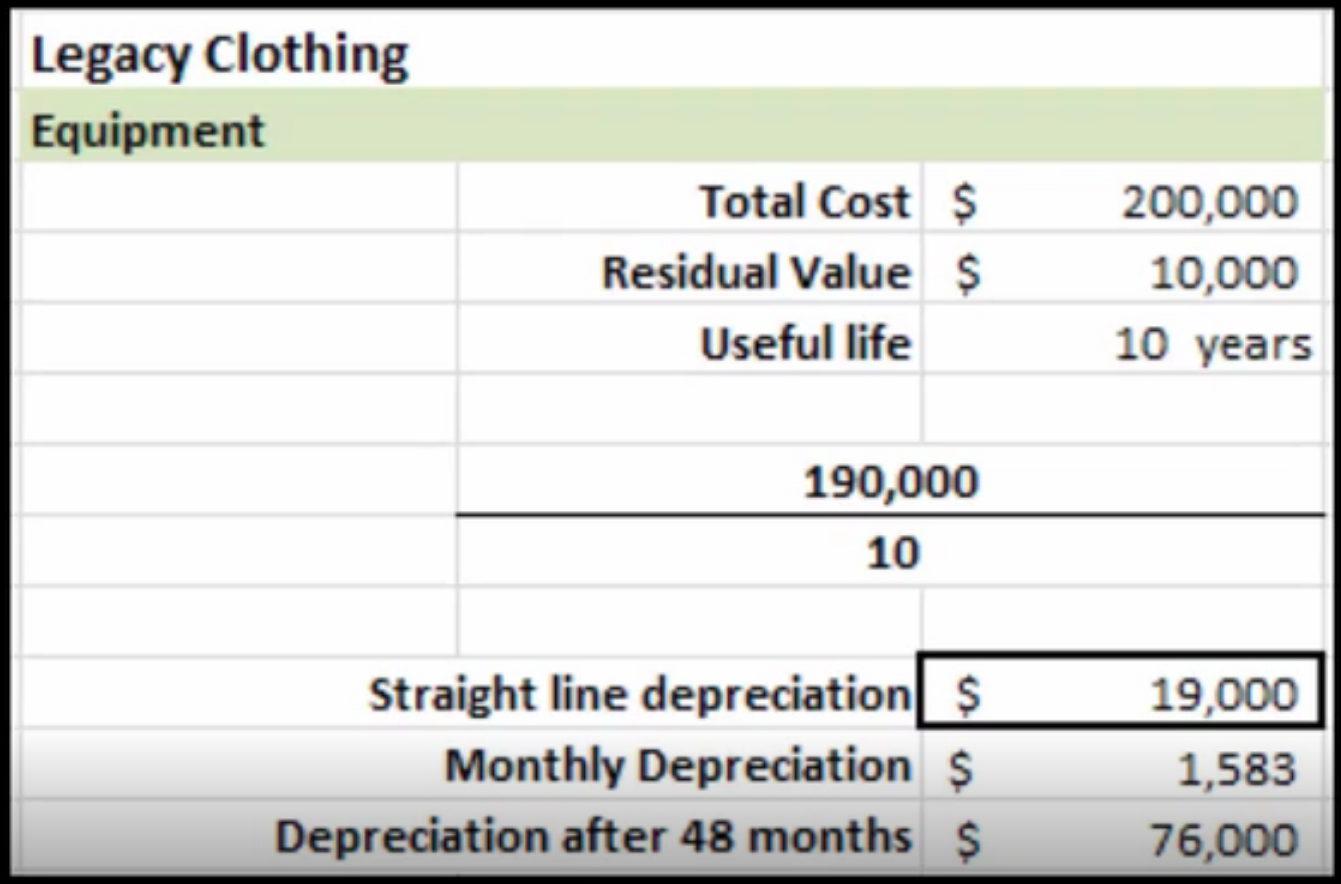## 3. Case Study: Double Declining Balance Depreciation

Now let's turn our attention to another method of depreciation, double declining balance. We need the same information as before: total cost, residual value, as well as the useful life.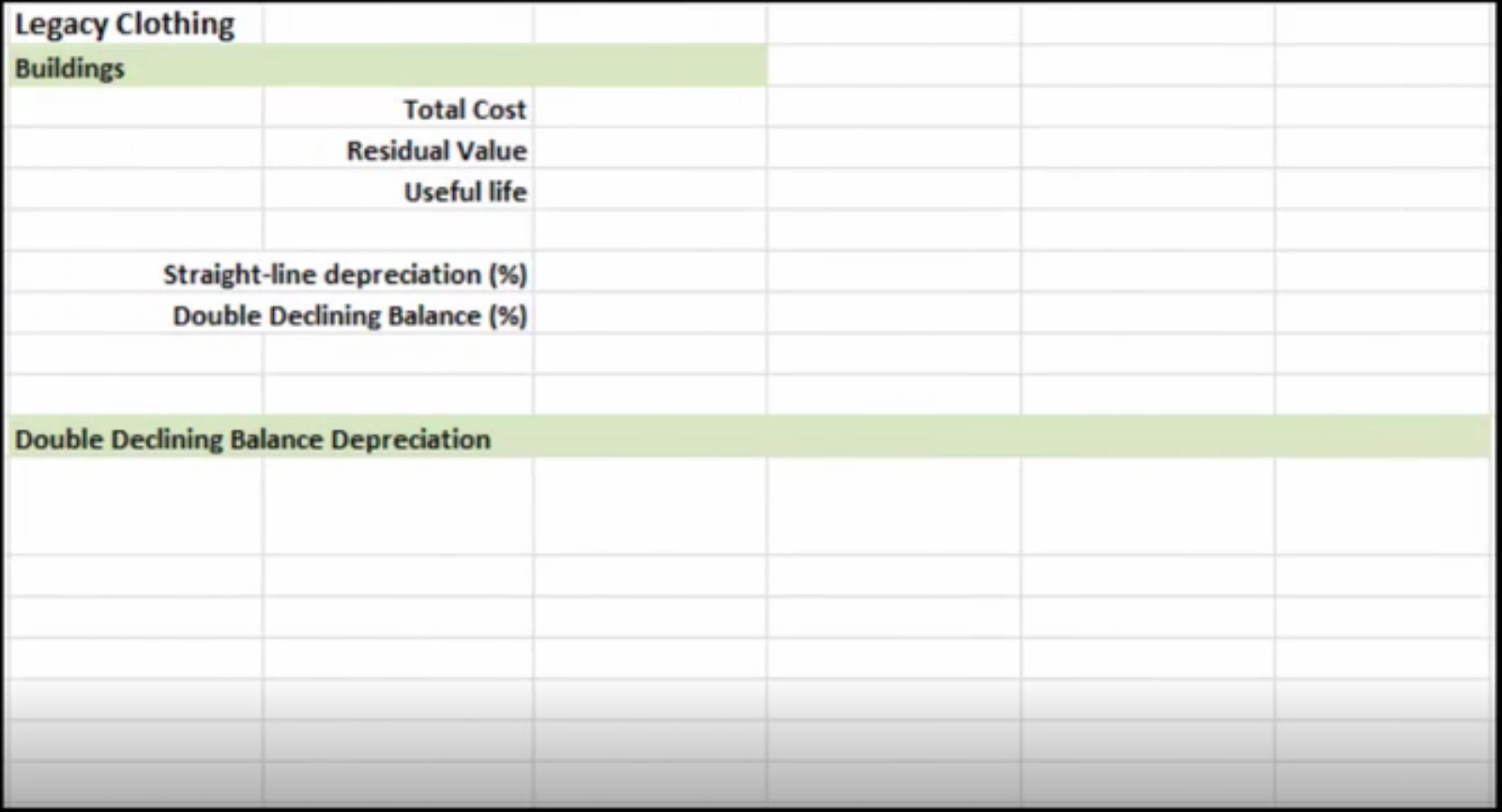3a. Buildings

Our buildings cost \$500,000 with a useful life of 30 years, so the straight line depreciation was \$16,667. Turning this into a percent, the straight line deprecation percentage is \$16,667 divided by \$500,000, or 3.33%. This means that every year, 3.33% of that asset would be depreciated.

Now, to calculate the double declining rate, we simply take that 3.33% straight line depreciation rate and multiply it by 2 to get a double declining balance percentage of 6.7%.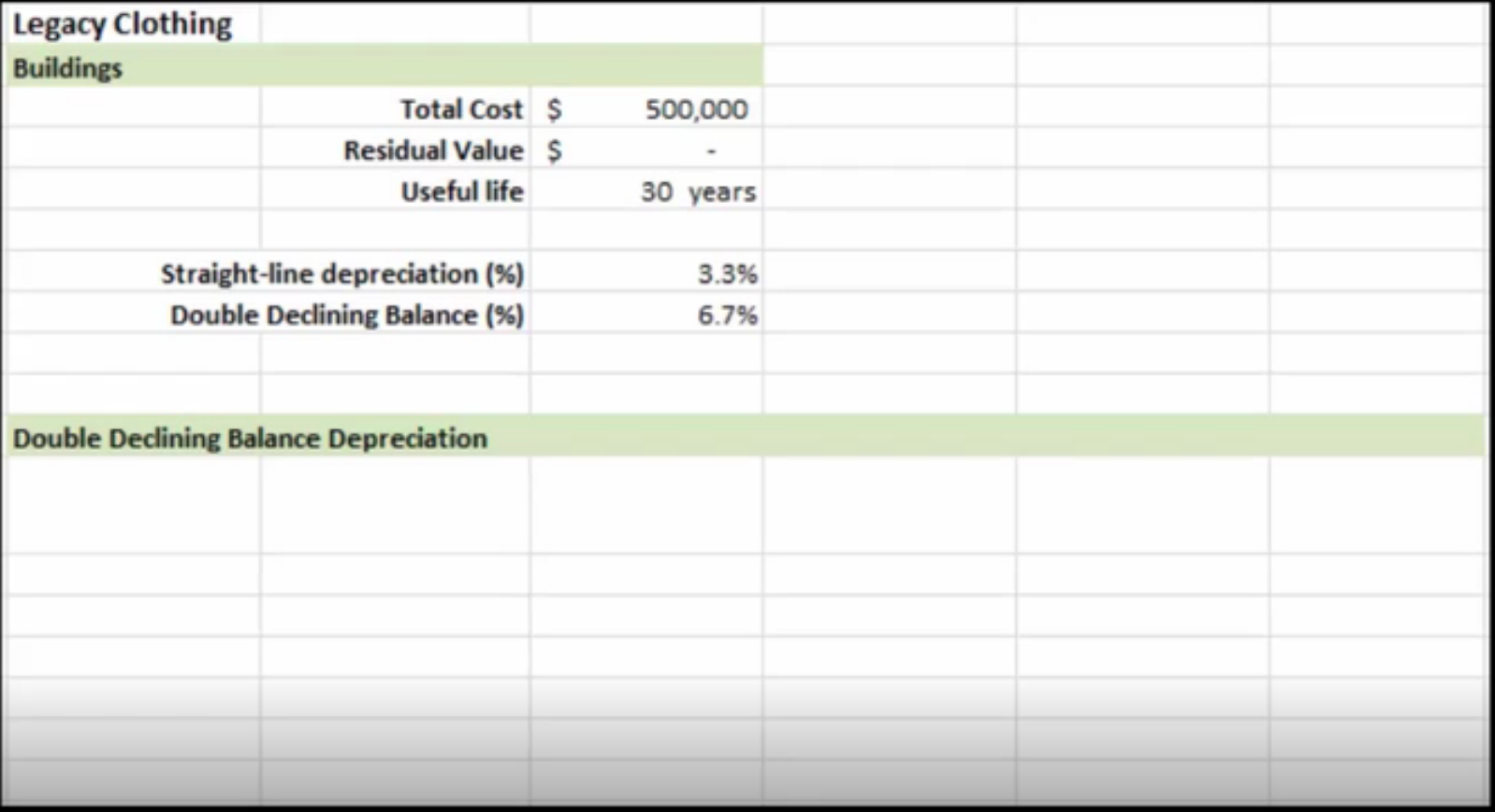Now we need to prepare our depreciation table, starting with the year. So, if we look at year one, beginning book value is 500,000. We have a depreciation rate of 6.7%, so our depreciation expense is \$33,333.

The book value at the end of the year, then, would be \$466,667.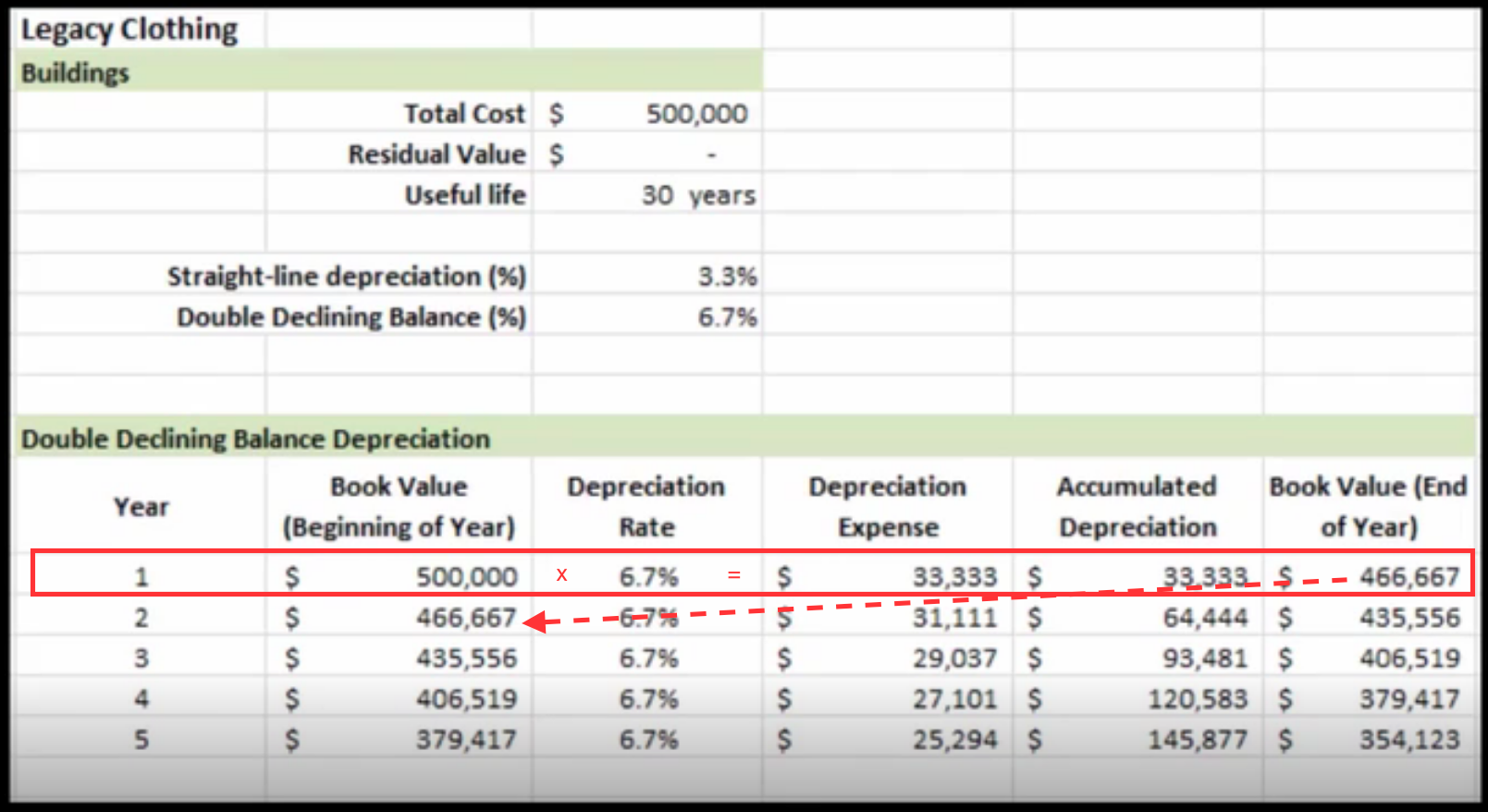Note that this ending book value of year one becomes our beginning book value in year two, and we go through the same process of multiplying it by the depreciation rate to get to the depreciation expense.

As you can see below, we add the current year's depreciation expense to the accumulated depreciation from the prior year to get the current year's accumulated depreciation.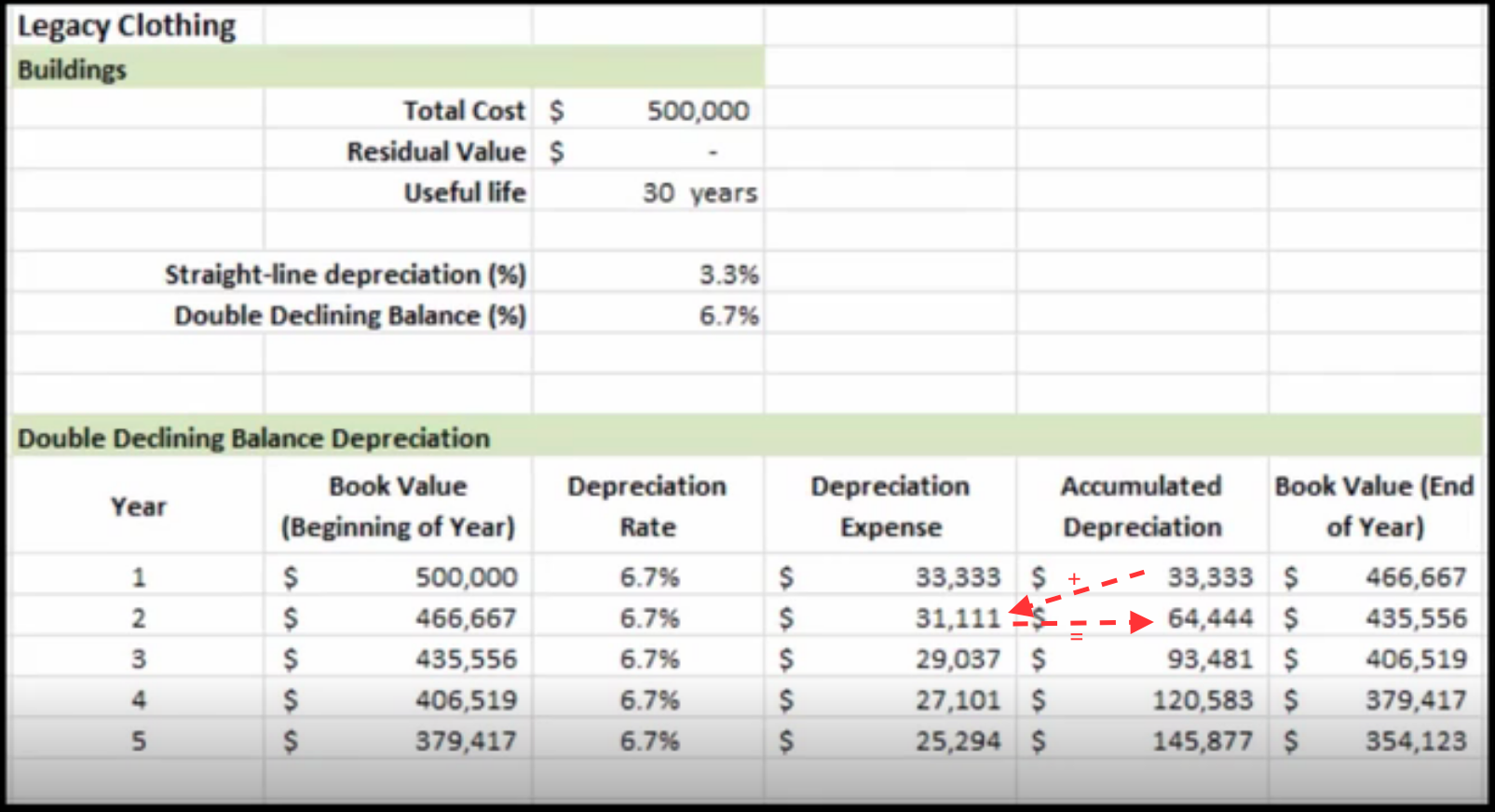Also, notice that in year 30, because this asset has a 30-year useful life and no residual value, we would record \$67,614 of depreciation expense in order to bring the book value at the end of 30 years to zero.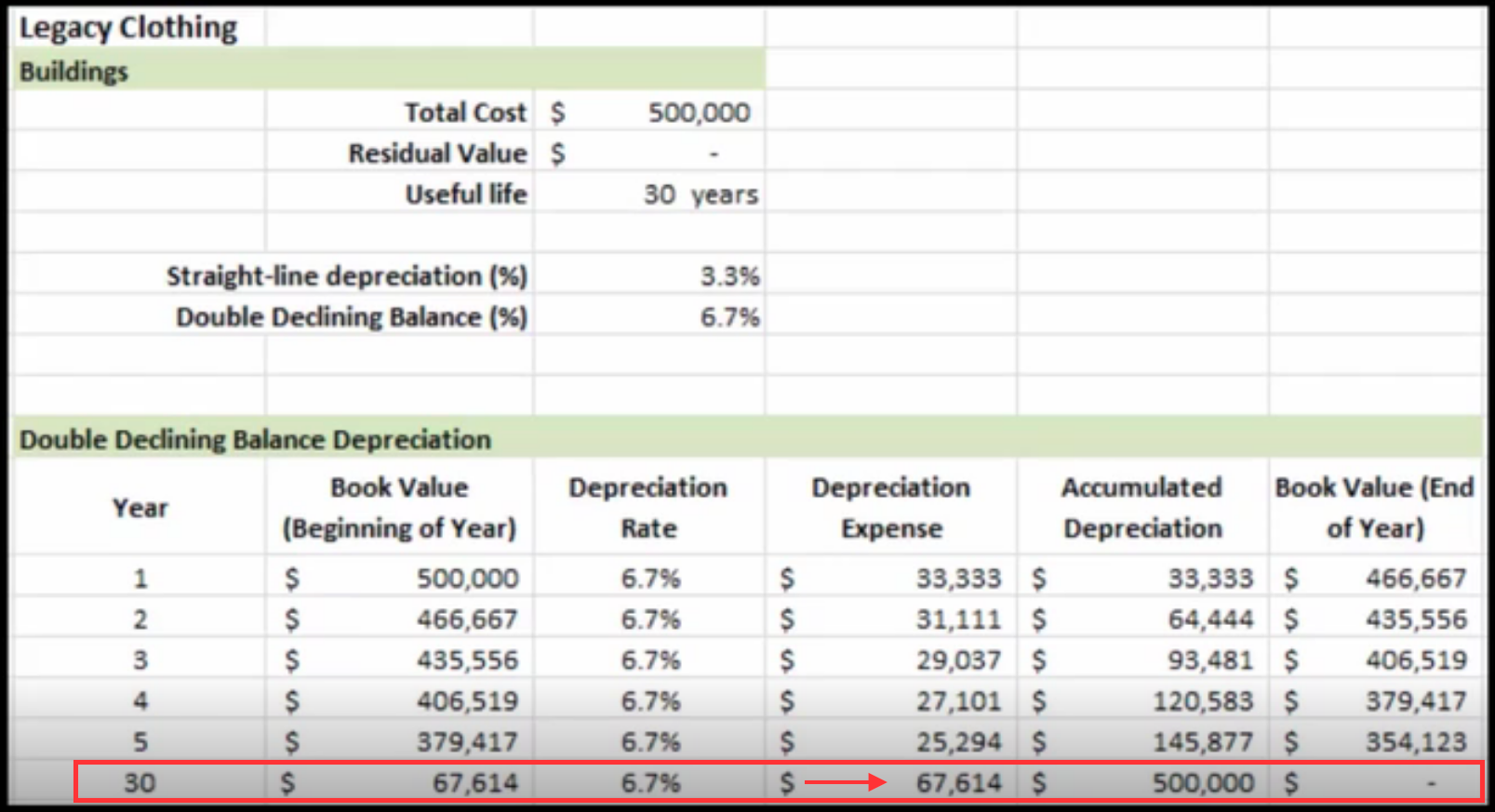3b. Equipment
Now we're going to do that same calculation for our equipment, and just like before, we need the total cost, residual value, and useful life.

We're going to use the same information as before, inputting a total cost of \$200,000, residual value of \$10,000, and a useful life of 10 years, which results in a straight line depreciation percentage of 10%. Therefore, 10% of this equipment is being depreciated every year under the straight line method.

Again, for double declining balance, we double that straight line depreciation rate to get 20%.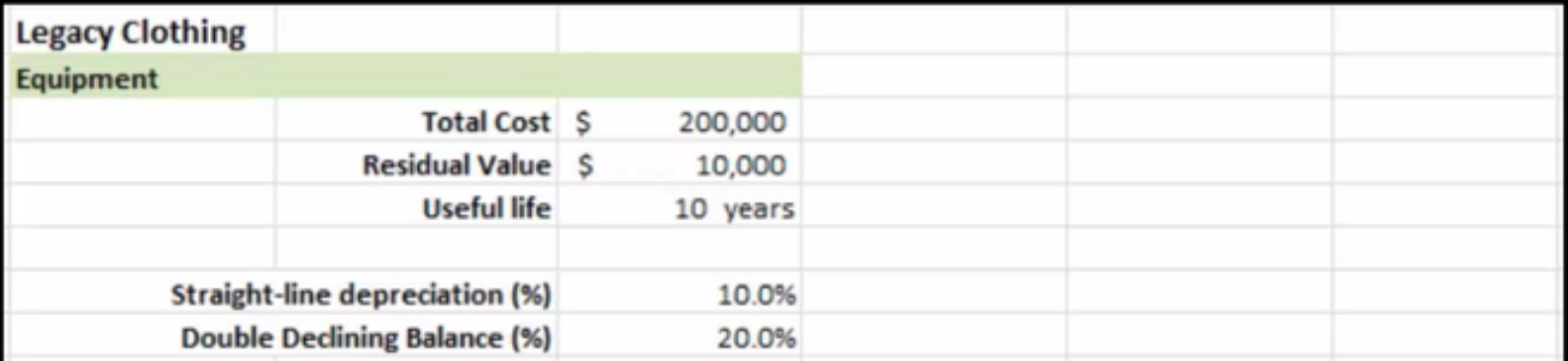Now we can populate our double declining balance depreciation table, going through the same process. In year one, we have a beginning book value of \$200,000, which is the equipment's cost.

Under double declining balance, we have a depreciation rate of 20%, so we multiply the book value by that depreciation rate to arrive at a depreciation expense of \$40,000.

Therefore, the book value at the end of year one is \$160,000, which becomes our beginning book value at the beginning of year two, and so on for each following year.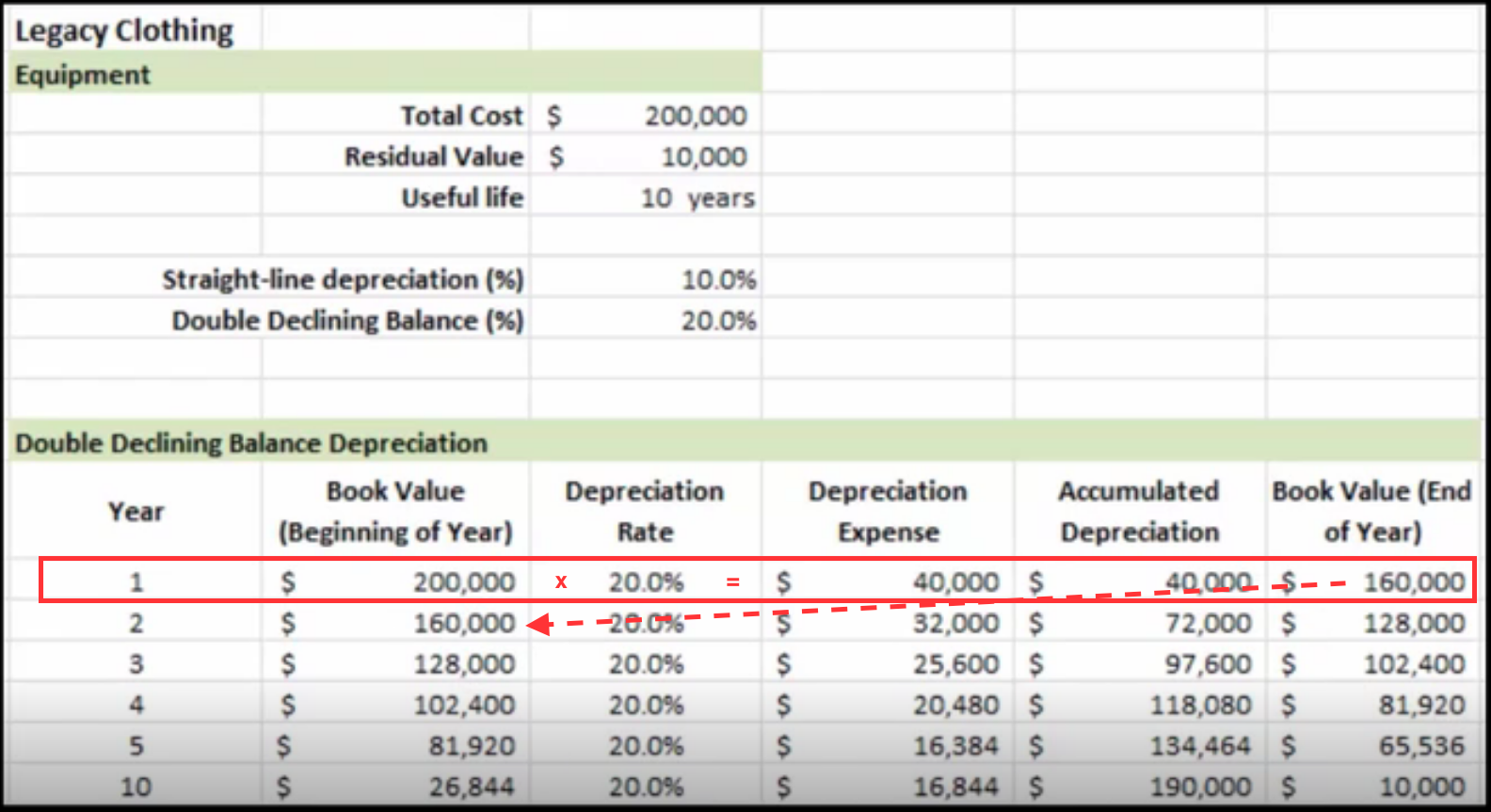Notice, though, what happens when we get down to year 10. Because this asset has a useful life of 10 years, we do not record 20% depreciation. Instead, we would record whatever depreciation gets us to \$10,000 residual value.

Because we are in the last year of the asset's useful life, we don't depreciate it to zero. We can only depreciate it to the residual value, but not beyond that point.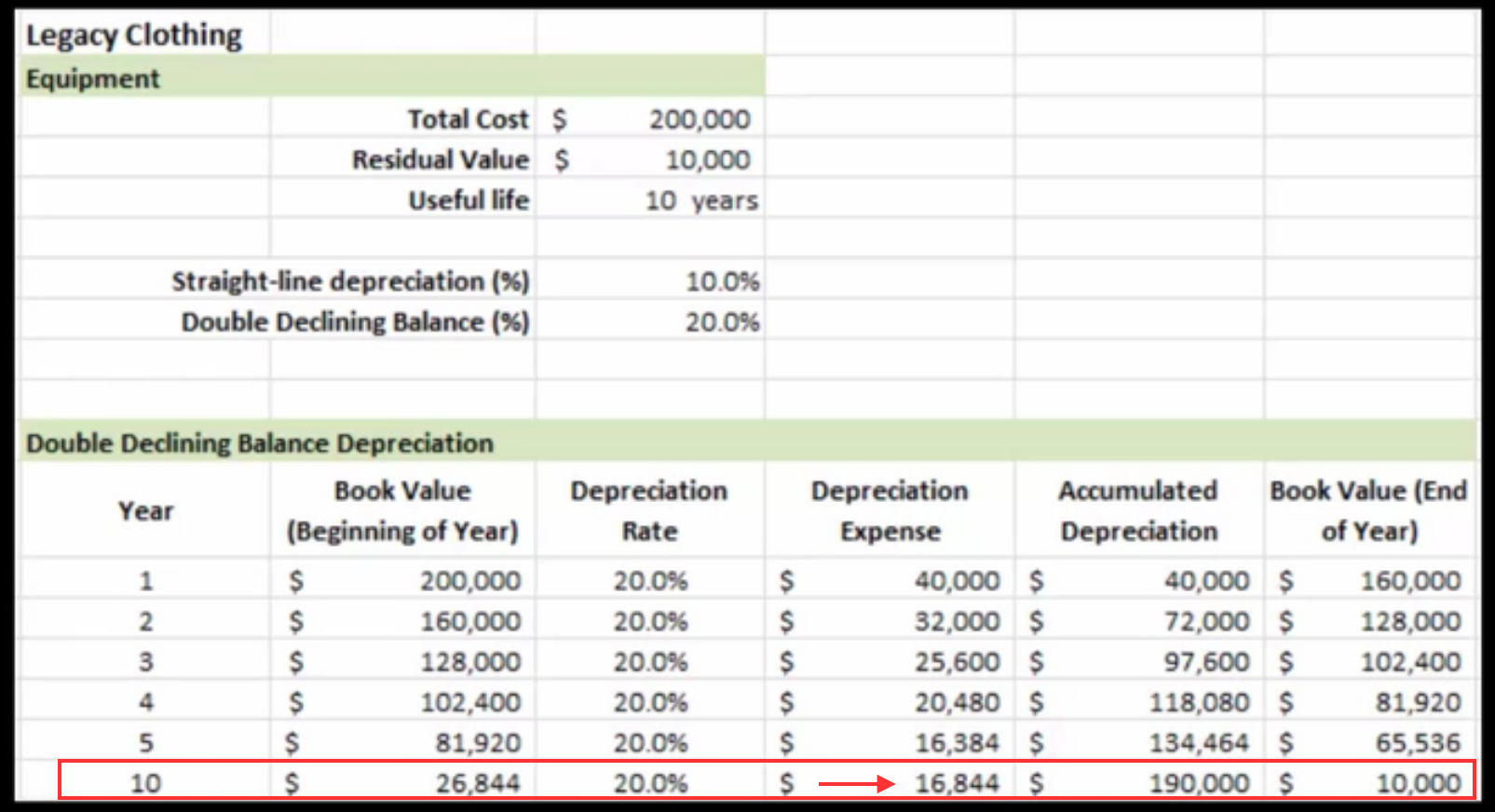summary
Today we introduced our case study company called Legacy Clothing, a department store selling men's, women's, and children's clothing and other related items. We explored examples of calculating depreciation on two fixed assets, buildings and equipment, using two methods: straight line depreciation and double declining balance depreciation.

Source: Adapted from Sophia instructor Evan McLaughlin.

Rating